﻿ 舱室内爆载荷特性研究
 舰船科学技术2020, Vol. 42Issue (1): 11-16PDF

Research on explosion load characteristics of cabin
YIN Qun, WANG Yi-ming, WANG Ke
School of Navy Architecture and Ocean Engineering, Jiangsu University of Science and Technology, Zhenjiang 212003, China
Abstract: Firstly, the ship model is selected and validated, and its model is simplified. The three-compartment finite element model in the middle of the ship is selected. The internal explosion process of the three-compartment model is simulated by nonlinear explicit dynamics software Dytran, and the Pressure, time and distance formulas are fitted by calculation, which are applied to the explosion as static load. The dynamic response of ship structure under internal explosion load is simulated by calculating the plate structure of the explosion chamber. Finally, the calculation results before and after simplification are compared and analyzed, and the feasibility of simplified calculation method of implosion load is judged by the calculation results of various parameters, which has important reference value for simplified calculation of explosion load in the future.
Key words: ship     numerical simulation     implosion load     simplified calculation
0 引　言

1 舱室内部爆炸过程数值模拟 1.1 材料模型表 1 舰船材料参数 Tab.1 Ship material parameters
1.2 有限元仿真模型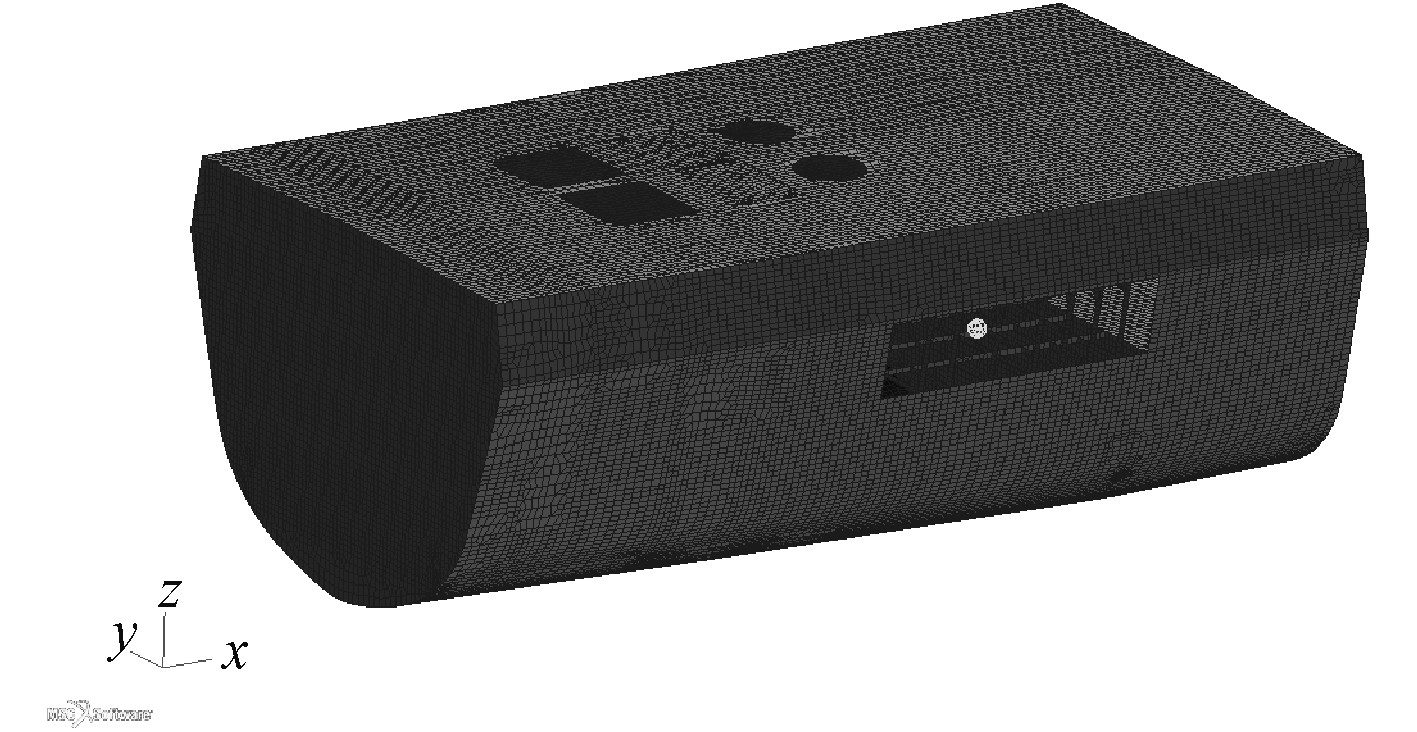图 1 有限元模型 Fig. 1 The model of finite element
2 舱室内部爆炸载荷特性研究 2.1 舱室内部爆炸冲击波参数计算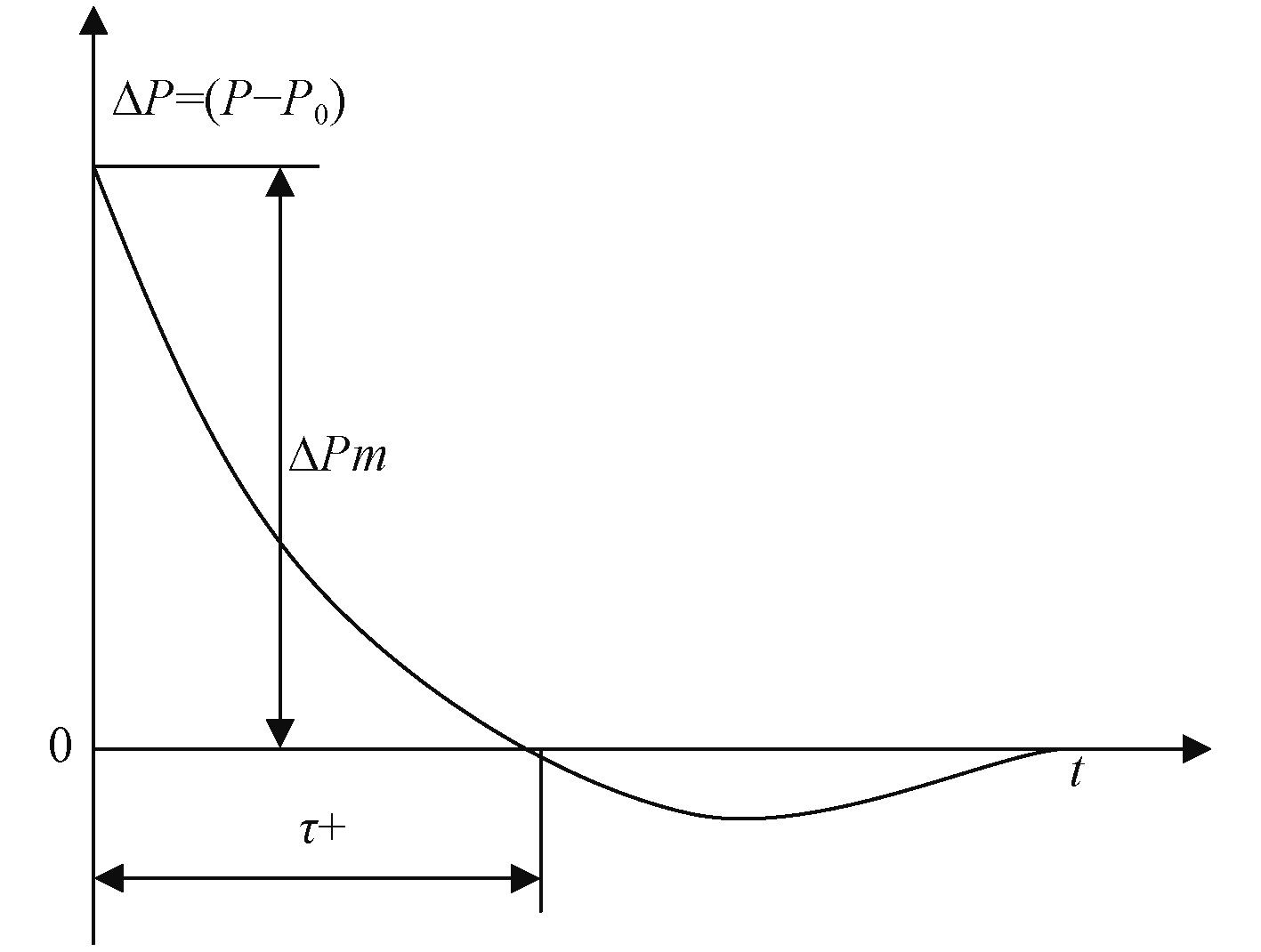图 2 有限元模型炸药位置示意图 Fig. 2 Sketch map of explosive location by finite element model

2.1.1 爆炸空气冲击波峰值超压 $\Delta {p_m}$ 的计算公式

CaдOBCKий得到

 $\Delta p = \frac{{0.076}}{{\bar r}} + \frac{{0.025}}{{{{\bar r}^2}}} + \frac{{0.65}}{{{{\bar r}^3}}}{\text{，}}$ (1)

Henrych得到

 $\Delta p = \frac{{1.379}}{{\bar r}} + \frac{{0.543}}{{{{\bar r}^2}}} - \frac{{0.035}}{{{{\bar r}^3}}} + \frac{{0.0006}}{{{{\bar r}^4}}}(0.05 \leqslant \bar r \leqslant 0.3){\text{，}}$ (2)
 $\Delta p = \frac{{0.6076}}{{\bar r}} - \frac{{0.032}}{{{{\bar r}^2}}} + \frac{{0.209}}{{{{\bar r}^3}}}(0.3 \leqslant \bar r \leqslant 1){\text{，}}$ (3)
 $\Delta p = \frac{{0.0649}}{{\bar r}} + \frac{{0.3973}}{{{{\bar r}^2}}} + \frac{{0.3226}}{{{{\bar r}^3}}}(1 \leqslant \bar r \leqslant 10){\text{。}}$ (4)

 $\Delta p = \frac{{0.082}}{{\bar r}} + \frac{{0.265}}{{{{\bar r}^2}}} + \frac{{0.686}}{{{{\bar r}^3}}}(1 \leqslant \bar r \leqslant 15){\text{。}}$ (5)

2.1.2 空气冲击波正压区作用时间 ${\tau _ + }$ 的计算

 \begin{aligned} {\tau _ + } = & {10^{ - 3}}\left( {0.107 + 0.444\bar R + 0.264{{\bar R}^2} - } \right.\\ & \left. {0.129{{\bar R}^3} + 0.0335{{\bar R}^4}} \right)\sqrt{W}{\text{。}} \end{aligned} (6)

2.1.3 空气冲击波比冲量 ${I_ + }$ 的计算

 ${I_ + } = \frac{C}{{\overline r }}\sqrt{{\tilde w}}{\text{。}}$ (7)

2.2 舱室内部爆炸载荷简化计算研究 2.2.1 舱室内部爆炸载荷特性研究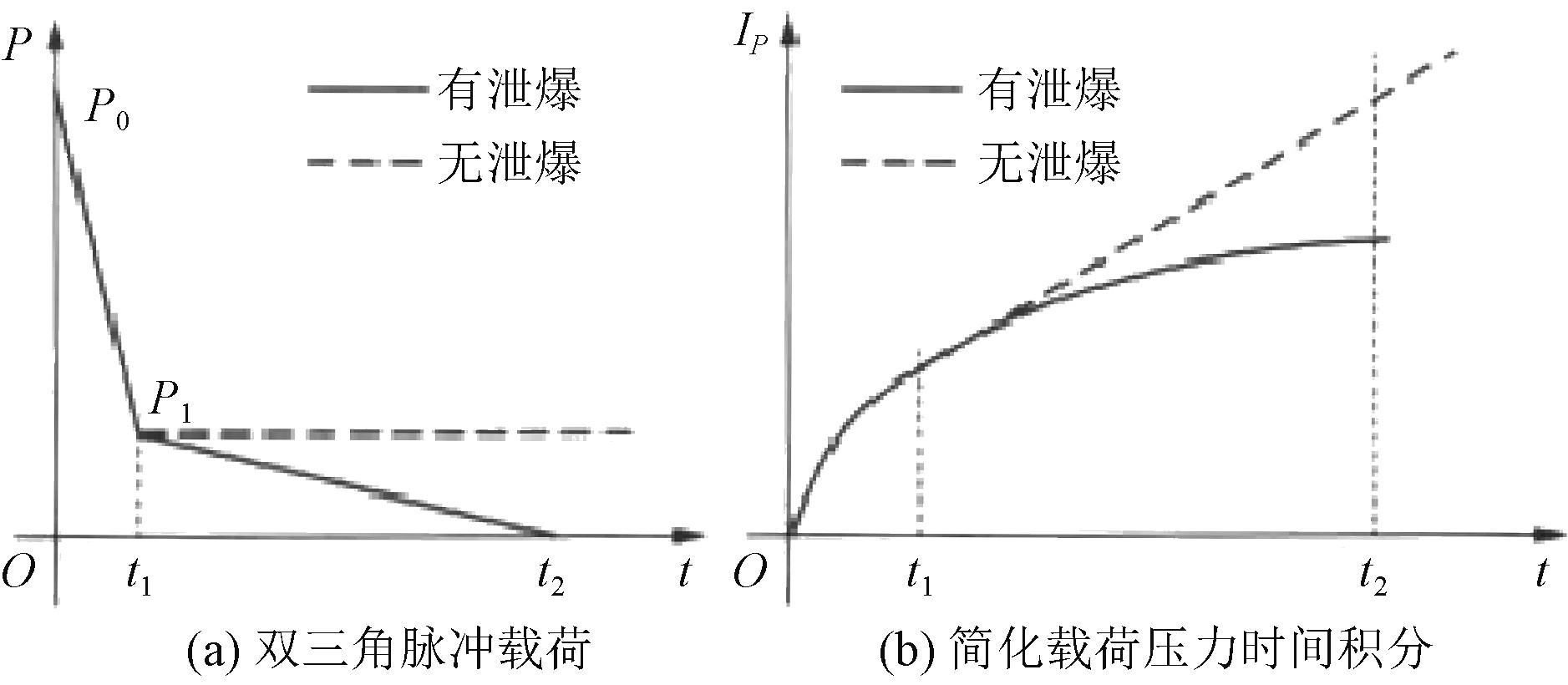图 3 结构物所受载荷特性 Fig. 3 Load characteristics of structures

2.2.2 舱室内部爆炸等效加载方法研究

 $\begin{split} P = & 19086206 - 356151.8 \times \\ & \sqrt{{\left( {{{(x - 68.5)}^2} + {{(y + 7.18277)}^2} + {{(z - 7.806)}^2}} \right.}}-\\ & \frac{{38481449.53}}{{\ln (\sqrt{{({{(x - 68.5)}^2} + {{(y + 7.18277)}^2} + {{(z - 7.806)}^2}}})}}+\\ & \frac{{195637319.1}}{{{{(x - 68.5)}^2} + {{(y + 7.18277)}^2} + {{(z - 7.806)}^2}}}{\text{，}}\\[-17pt] \end{split}$ (8)
 $P = 3778.062 \times {e^{( - 15497.5 \times t + 689.2734 \times \sqrt t )}} + 48874227 \times t{\text{。}}$ (9)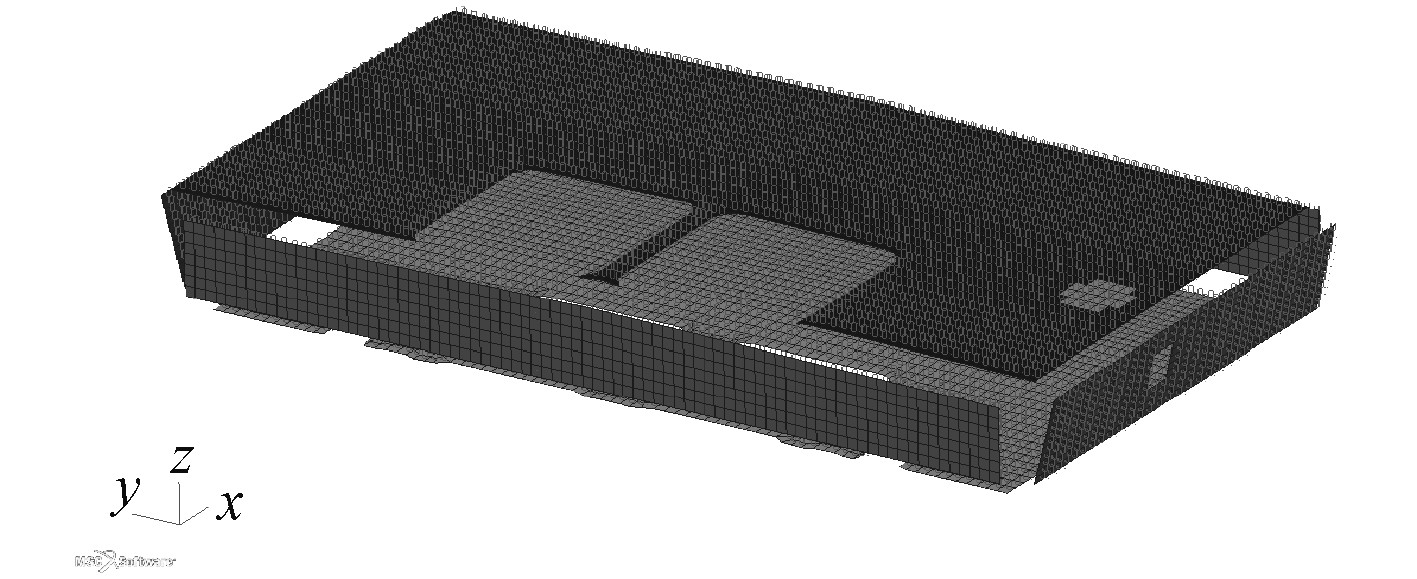图 4 一倍Pressure加载单元示意图 Fig. 4 Schematic diagram of double Pressure loading unit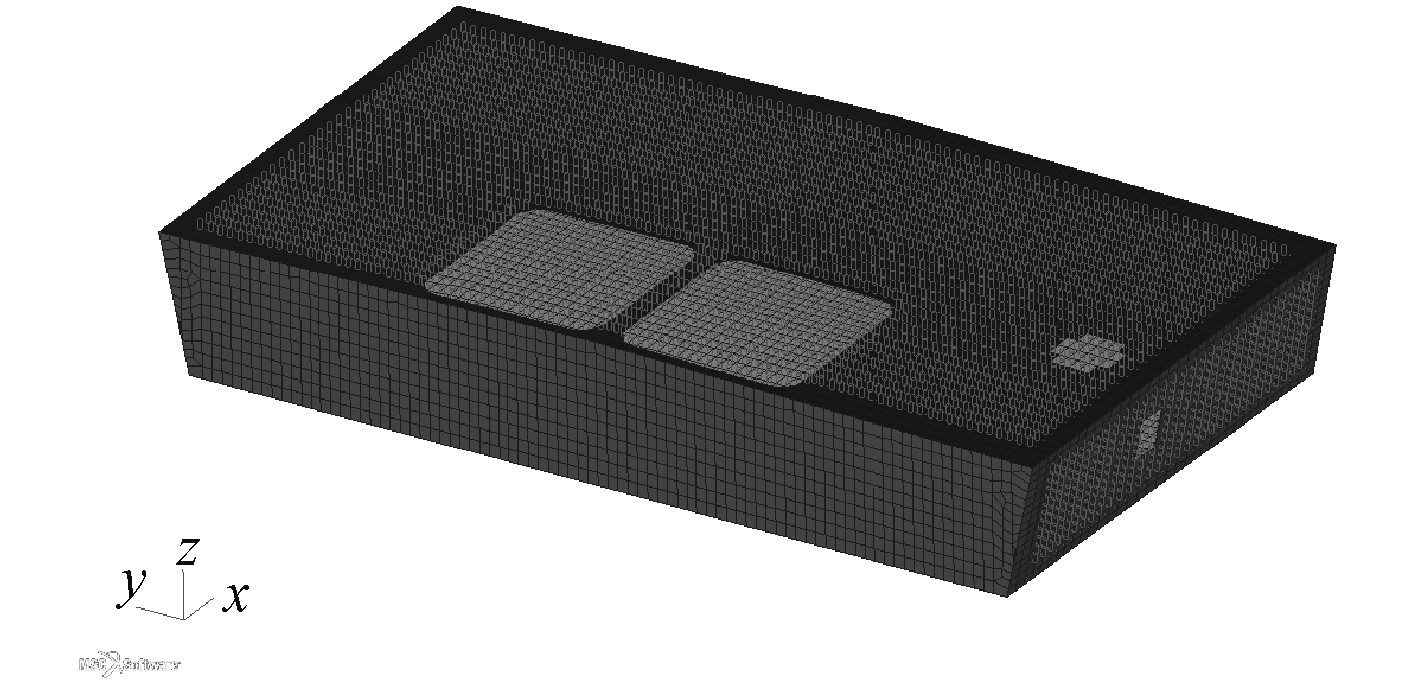图 5 一倍Pressure在爆炸舱室加载区域示意图 Fig. 5 Schematic diagram of loading area of double Pressure in explosive chamber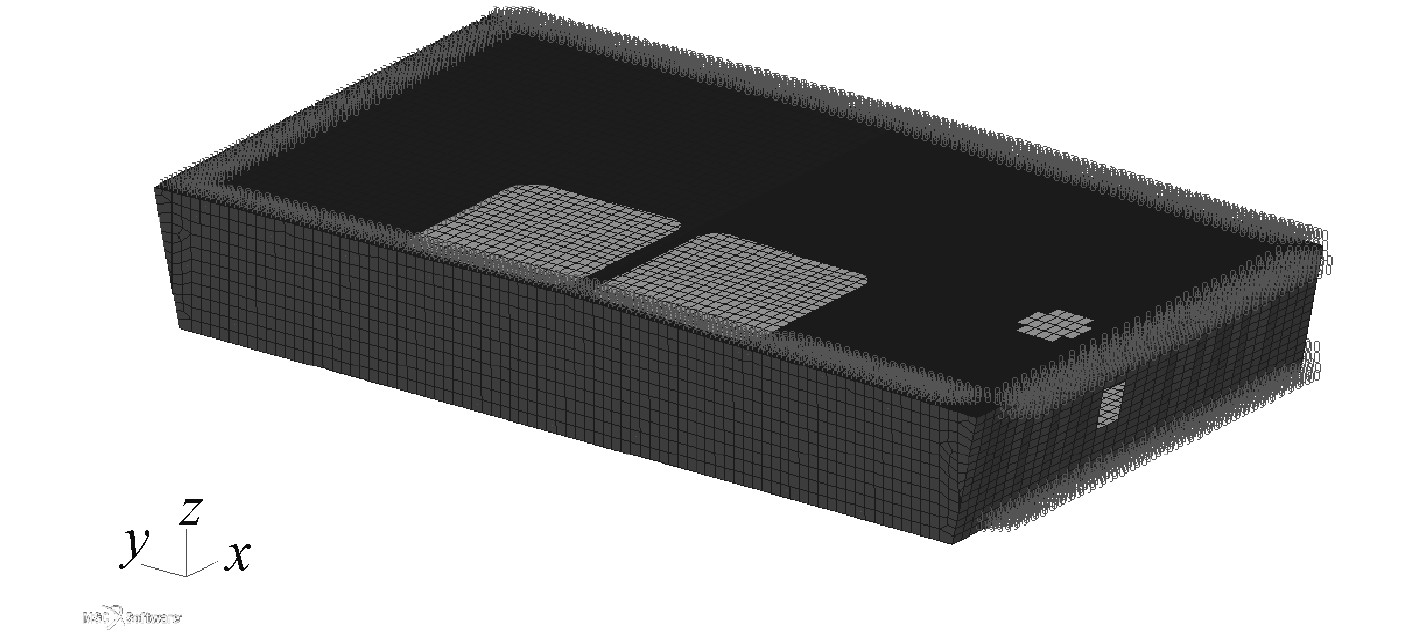图 6 四倍Pressure在爆炸舱室加载区域示意图 Fig. 6 Schematic diagram of loading area of four times Pressure in explosive chamber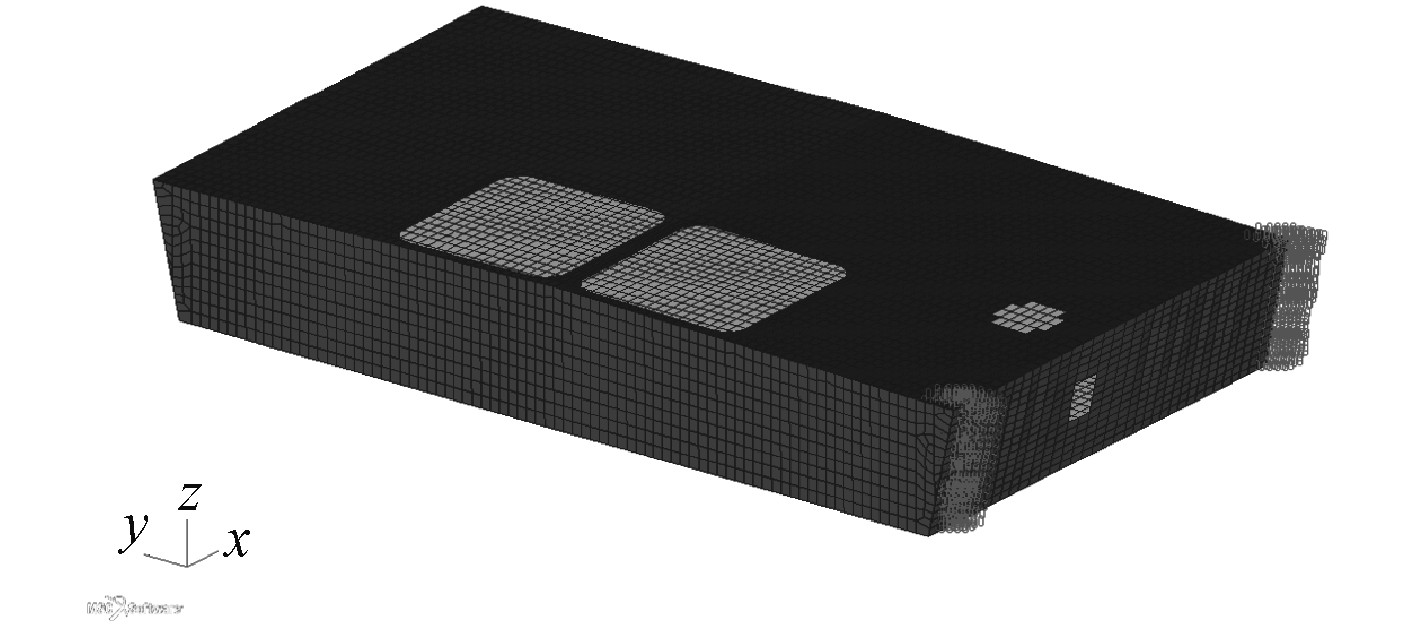图 7 八倍Pressure在爆炸舱室加载区域示意图 Fig. 7 Schematic diagram of loading area of eight times pressure in explosive chamber
3 计算结果分析比较

3.1 爆炸舱室结构的动态响应对比分析

3.2 炸舱室结构的应力对比分析表 2 应力计算结果对比图 Tab.2 Comparison diagram of stress calculation results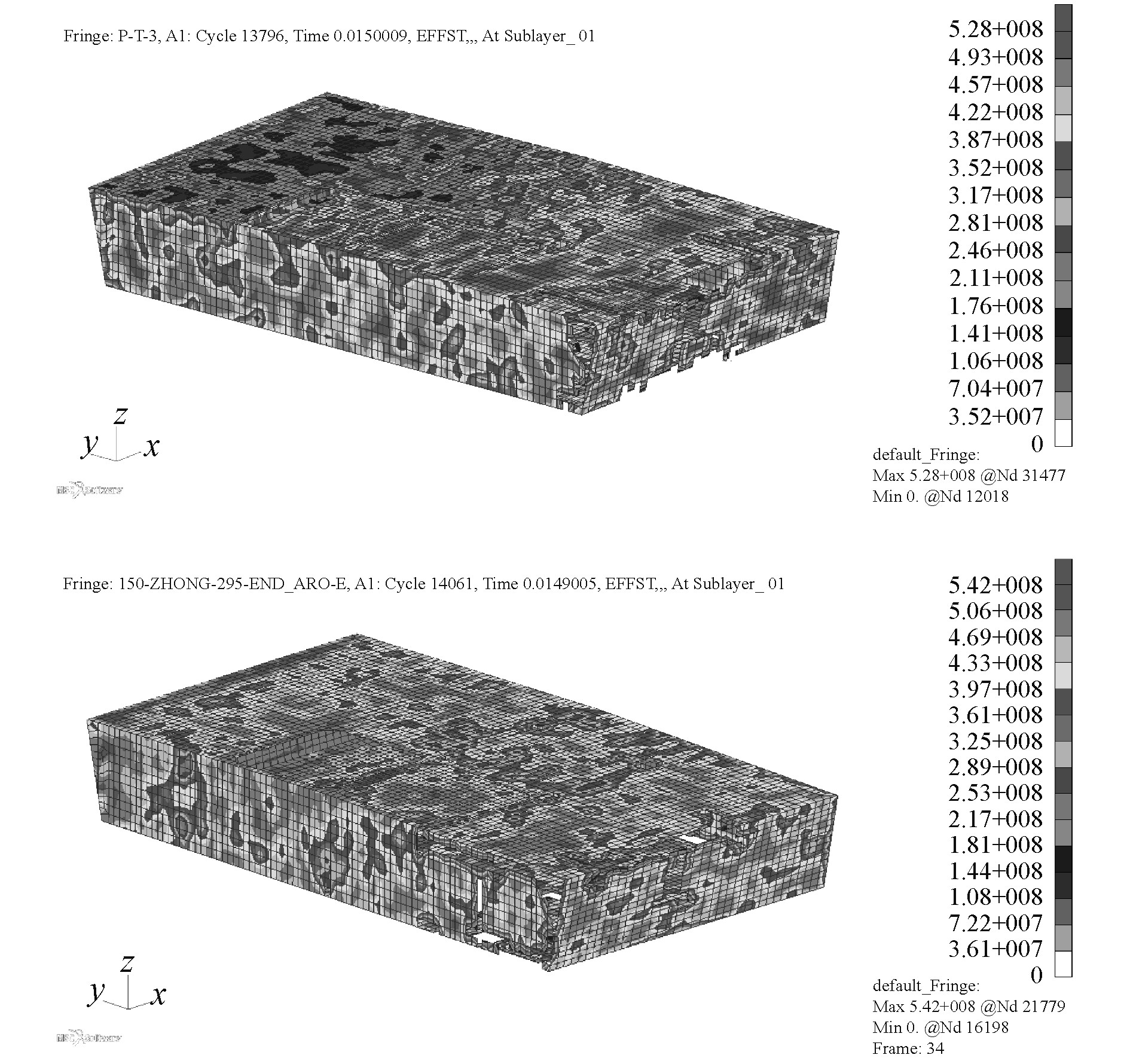图 8 爆炸舱室应力对比图 Fig. 8 Stress compartments for explosive compartments
3.3 炸舱室结构的应变对比分析表 3 应变计算结果对比图 Tab.3 Comparison diagram of strain calculation results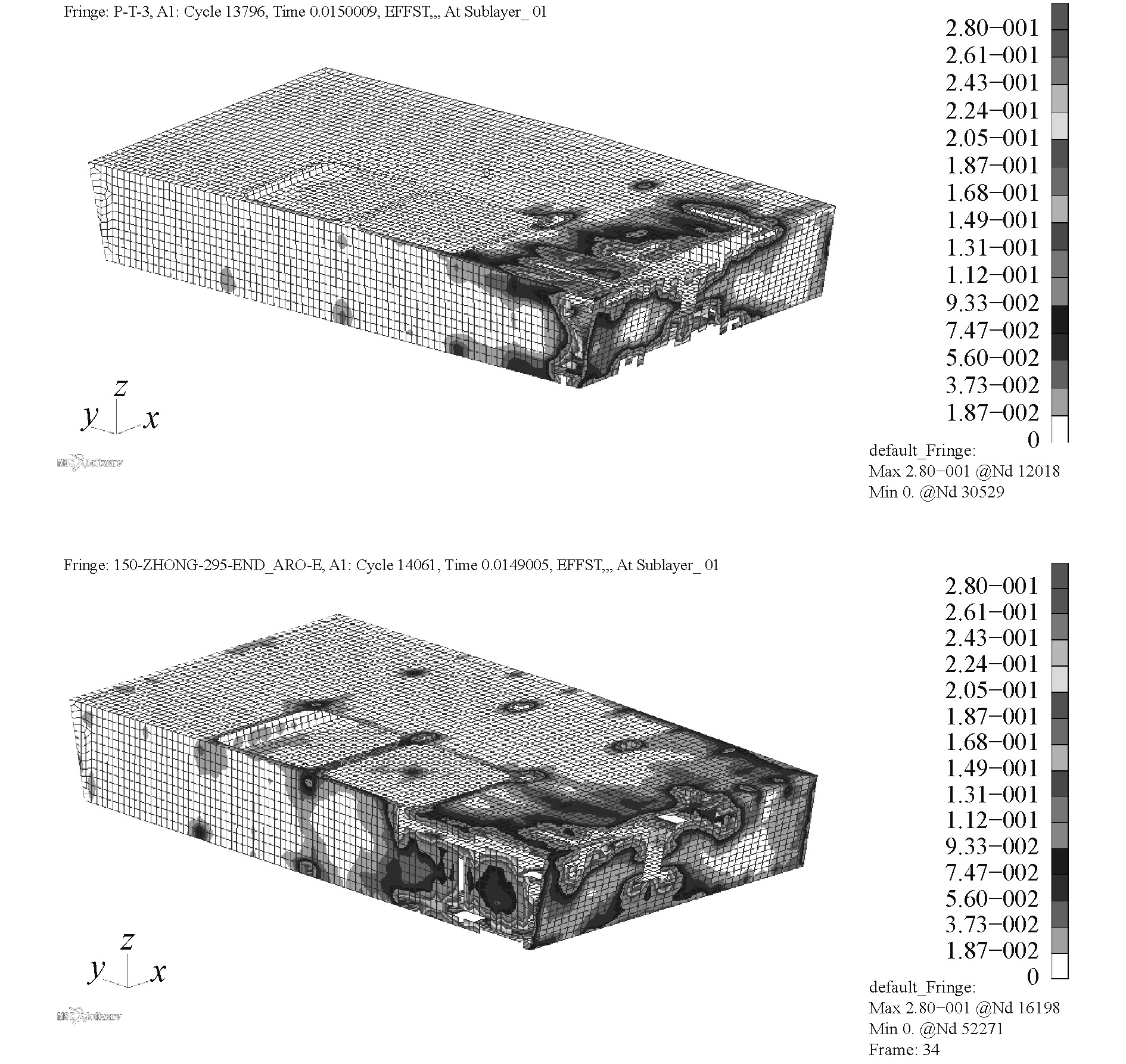图 9 爆炸舱室应变对比图 Fig. 9 Comparison diagram of strain in explosion compartments
3.4 炸舱室结构的位移对比分析表 4 位移对比图 Tab.4 Displacement contrast map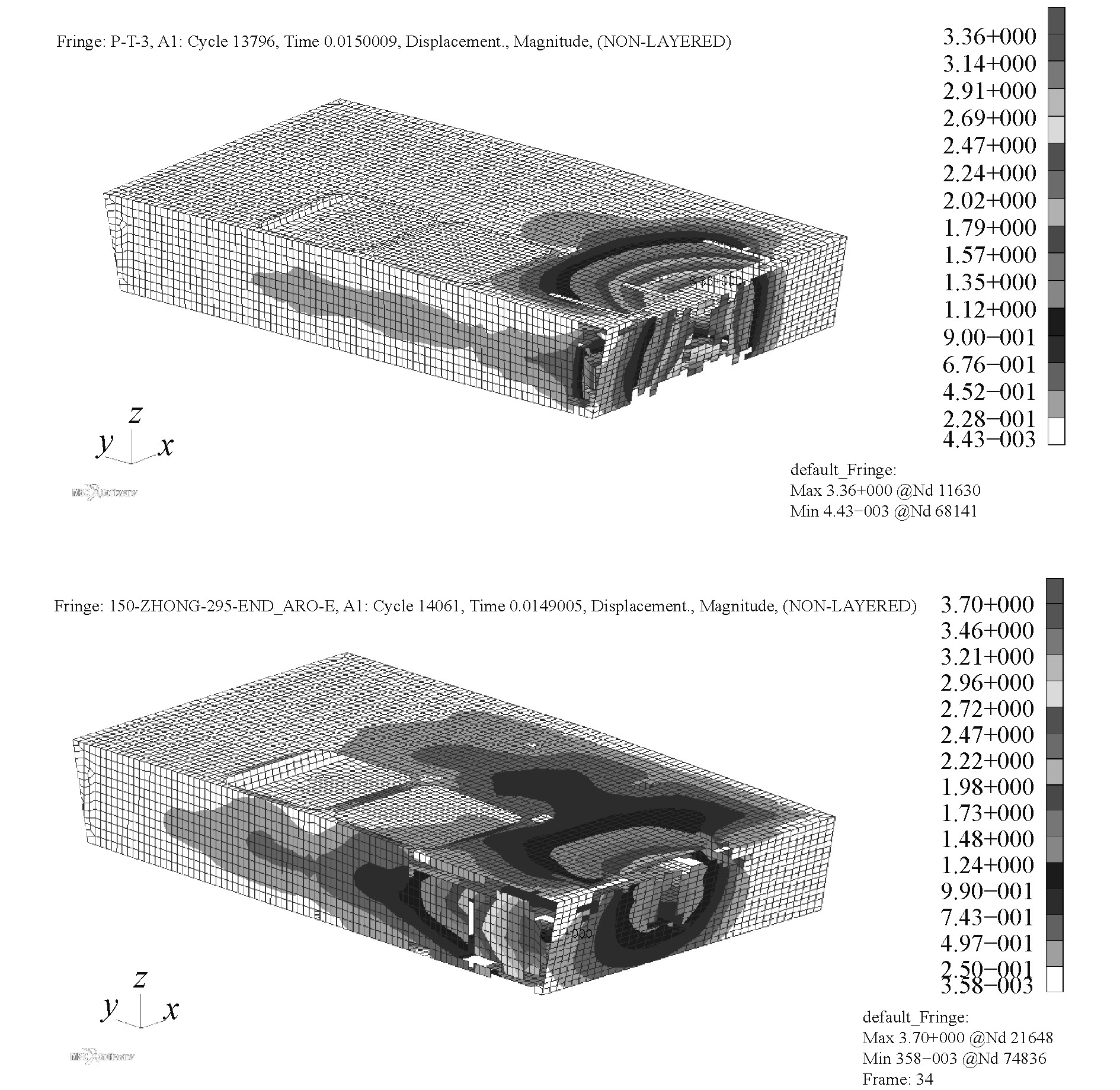图 10 爆炸舱室应变对比图 Fig. 10 Comparison diagram of strain in explosion compartments
3.5 炸舱室结构的破口对比分析表 5 爆炸毁伤对比 Tab.5 Comparison map of explosion damage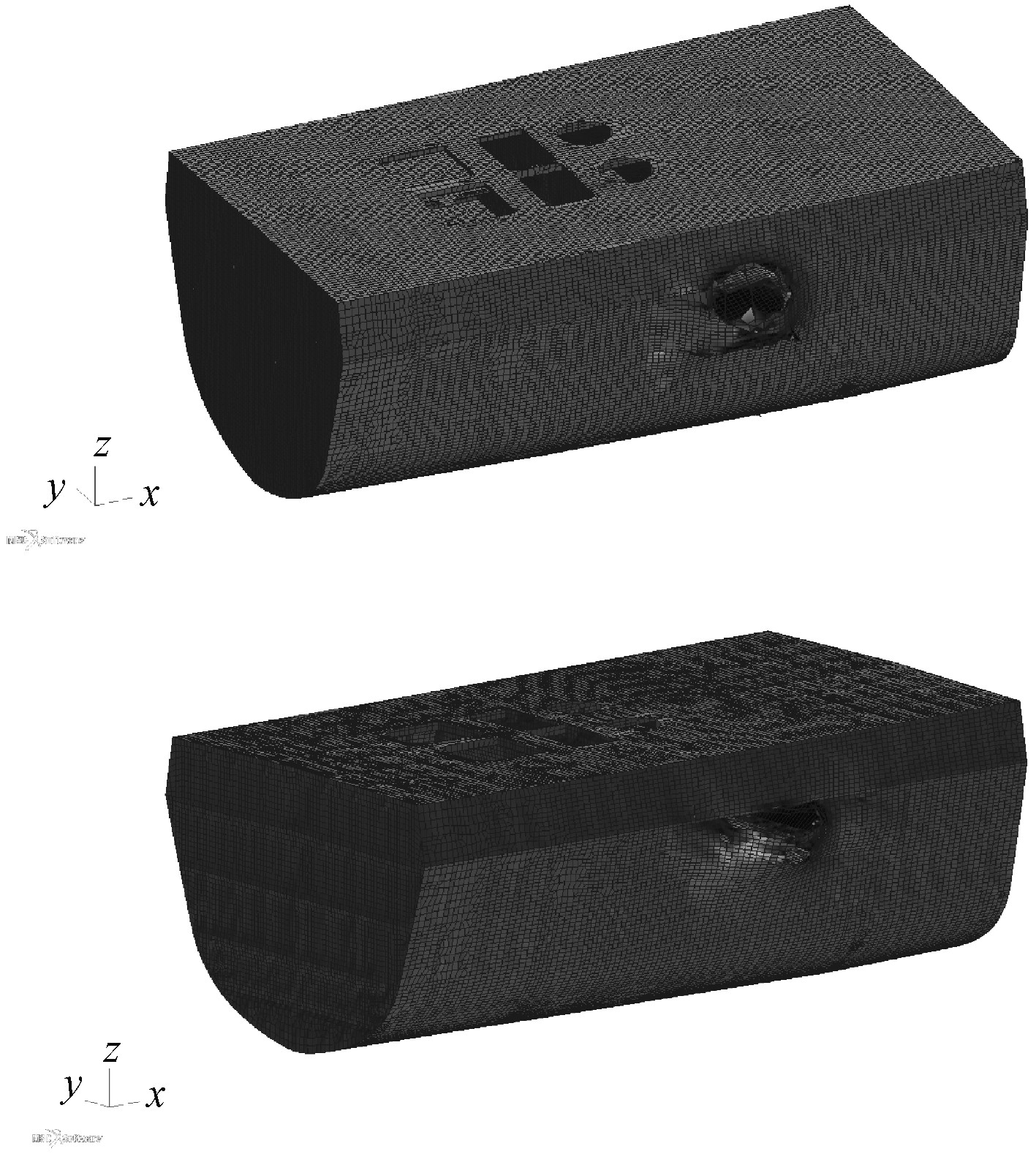图 11 破口对比图 Fig. 11 Breach contrast map
4 结　语

1）舱室内部爆炸时，首先冲击波会作用到最近的板架结构，并随着进一步的传播在板架连接处和角隅处汇聚、反射，对舰船结构造成进一步的毁伤；

2）由数值仿真和各种计算结果的比较分析可以发现，无论是从应力、应变、位移及破口方面考虑，内爆载荷简化计算方法的误差最大不超过10%，始终控制在误差允许的范围之内，并能够节省大量的计算时间和计算成本，具有一定的可行性，为后续计算提供了简化计算方法。

  朱锡, 张振华, 梅志远, 等. 舰船结构毁伤力学[M]. 北京: 国防工业出版社, 2013.  张克咏. 爆炸冲击波作用下舰船新型舱壁结构型式及响应预报研究[D]. 镇江: 江苏科技大学, 2015.  宁建国, 王成, 马天宝. 爆炸与冲击动力学[M]. 北京: 国防工业出版社, 2010.  HENRYCH. J. The Dynamic of Explosion and its use[M]. Printed in Czechoslovakia, 1979.  张挺. 爆炸冲击波测量技术(电测法)[M]. 北京: 国防工业出版社, 1984.  杨科之, 杨秀敏, 王年桥. 解放军理工大学学报(自然科学版)[J]. 解放军理工大学, 2002(4): 31-33.